# Interior Lighting Design Methods- Part One

I indicted before in our course "
, that we can design interior lighting by using any method from the following three ones:

1. The Zonal Cavity (Lumen) method,
2. Point by point method,
3. Watt per square foot method.

Today I will explain the first method for interior lighting design as follows.

You can review the following previous articles for more information and good following:

First: The Zonal Cavity (Lumen) method

• The zonal cavity, or lumen, method is used to calculate average illuminance.
• It is used when there is uniform lighting with either incandescent, fluorescent, or HID lighting systems.
• The illuminance at the center of the room can be assumed to be uniform, as indicated by the average illuminance values derived from the zonal cavity formula.
• The method is based on the assumption that you can divide the room into three cavities as follows:
1- Ceiling cavity

It is the distance from bottom of luminaires to the ceiling.

Ceiling cavity = zero if luminaires are surface mounted or recessed as indicated in (fig.1).

2- Room cavity

It is the distance from bottom of luminaire to the work surface.

The height of work surface for different activities will be assumed as follows:

• 30" for offices.
• 36 to 38" for counters, drafting, etc.
• 40 to 48" for stores, shops.
• Zero (floor level) for corridors, carpet stores.

3- Floor cavity

It is the distance from bottom of work surface to floor.
there is no floor cavity if room cavity is to the floor).

Zonal cavity (Lumen) method calculations by using IES Procedures:

IES( Illuminating Engineering Society) had been developed a procedure for calculation of average illuminance by using The Zonal Cavity (Lumen) method which is the best and more professional one between other procedures for doing the same.
Zonal cavity (Lumen) method calculations by using IES Procedures include (11) steps plus the spacing criteria as follows:

Step#1: Determine the generic “Type of Activity” to determine its “Illuminance Category”.  (see fig.2)Fig.2

Step#2: determine the “Range of Illuminance” from the chart of IESNA illuminance Recommendations or other standards. (see fig.2)

Step#3: Using Table a. or b. in fig.3, determine the weighting factors applicable to the illuminance category selected.Fig.3

Add the weighting factors algebraically. If the sum is -2 for Categories A-C or -3 for Categories D-I, use the lowest of the three illuminance values; if +2 or +3 for the same respective category ranges, use the highest illuminance value. Otherwise use the middle illuminance value.

Example#1

what is the weighting factor and Illumination level for category E in fig.1 above, noting the following:

• Age - 56
• Importance of speed and accuracy - (important)
• Background reflectance - (medium contrast, about 40%)

Age - 56:  then , factor +1

Importance of speed and accuracy - (important): then,factor 0

Background reflectance - (medium contrast, about 40%): then,factor 0

Total sum of factors = +1

In accordance with 'Table 1b' instructions, we use a weighting factor of +1 and then select the middle value of 75 footcandles for the task.

Step#4: select a suitable lighting luminarie from the manufacturer’s catalogs for the activity and place under design.

" for more information about how to select the right luminaire for a given application or activity,

Step#5: Calculations of Room, Ceiling and Floor Cavity Ratios (see fig.4)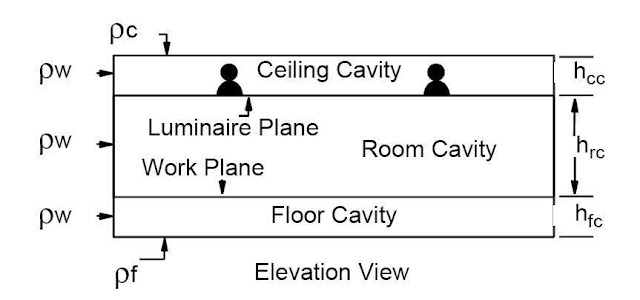Fig.4

1- the Room Cavity Ratio will be calculated as follows:and For Square Rooms, it will be:and For Irregular Shaped Rooms
, it will be: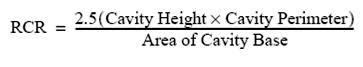2- Ceiling Cavity Ratio (CCR)
will be calculated as follows: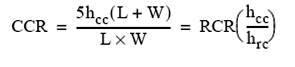3- Floor Cavity Ratio (FCR)
will be calculated as follows:Note: For square rooms or irregular rooms calculate RCR then use the ratio of the cavity heights to determine CCR and FCR.

Where:
RCR = Room Cavity Ratio

CCR = Ceiling Cavity Ratio

FCR = Floor Cavity Ratio

W = Room Width

L = Room Length

hcc = height of ceiling cavity. For surface mounted or recessed fixtures hcc = 0

hfc = height of floor cavity

hrc = height of room cavity

Example#2 :

In an office space 3m x 4.6m with a 2.6m ceiling height, Determine the room cavity ratio Assuming a workplane height of 0.76m (typical desk height).

Room cavity height (hRC) = Luminaire height – Workplane height
hRC = 2. 59 m – 0.76m = 1.83m
the luminaires are recessed in the ceiling so the luminaire height is the as the ceiling height. Computing the room cavity ratio, we have:
RCR = 5 x Room cavity height (Length + Width)/ (Length x Width)

RCR = 5 x 1.83m (3.05m + 4.57m)/ (3.05m x 4.57m)

RCR = 5
Then, CCR = zero.

And, FCR = RCR (hfc / hrc) = 5 (0.76/1.83)=2.1

Step#6: Determination of Room surface reflectances

This method assumes that you can determine the reflectance of the major surfaces of the room. Two relectance will be determined the actual and the effective reflectances as follows:

1- Cavity Surface Reflectance

It is the actual reflectance of the surface that is opposite the opening to the cavity. So, we have three values as follows:

• rc = Ceiling
• rw = Walls
• rf = Floor

2- Effective Cavity Reflectances

It is the combination of actual reflectances within a given cavity into one reflectance at the face of the cavity. So, we have two values as follows:

• rcc = Effective Ceiling Cavity Reflectance
• rfc = Effective Floor Cavity Reflectanc

You must determine the ceiling,wall, and floor reflectance values from the available tables of different organization standards like IESNA and others.
Table for typical approximate reflectances are as follows: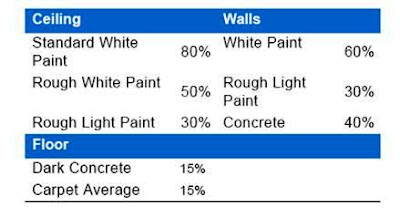Note: The general application of the procedure assumes no furniture present. It is possible to calculate this by taking average reflectance of all furniture times its total area then average with remainder of floor surface reflectance (and wall reflectance).

Step#7: Determination of Coefficient of Utilization (CU)

Factors influencing coefficient of utilization:

1. The efficiency of the luminaire,
2. The luminaire distribution,
3. The geometry of the space,
4. The reflectances of the room surface.

Knowing the ceiling, walls and floor reflectances and the room cavity ratio, the coefficient of utilization may be found from the manufacturers published data or typical data found in the current IESNA Handbook.

Example#3:

with reference to fig.5 , For a room cavity ratio of 1.0 and reflectances of 30 (ceiling), 30 (wall), and 20 (floor). determine the CU for this luminaire.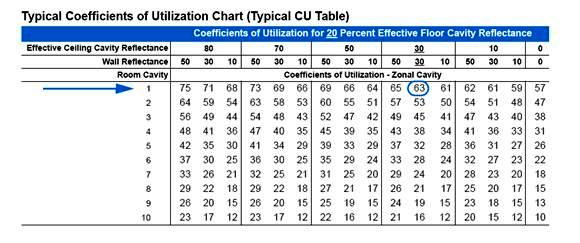Fig.5

CU = 0.63 which means that 63% of the lumens given off by the lamps reach the workplane and the other 37% are absorbed by the luminaire or the room surfaces and never reach the workplane.

Example#4:

Continuing with Examples #2 , the following assumptions are made after consulting the IESNA Lighting Handbook Table on Effective Reflectances:

• Effective Ceiling Cavity Reflectance, ρCC = 0.70
• Wall Reflectance, ρW = 0.50
• Effective Floor Cavity Reflectance, ρFC = 0.20
determine the CU for this luminaire.

RCR = 5 (calculated in example# 1)

Then,
consulting the IESNA Lighting Handbook, you will find that CU = 0.50,

Note:

Each luminaire has its own CU table specific to that luminaire’s light distribution and efficiency (see fig.6). CU values are listed in tables for different room geometries and room surface reflectances.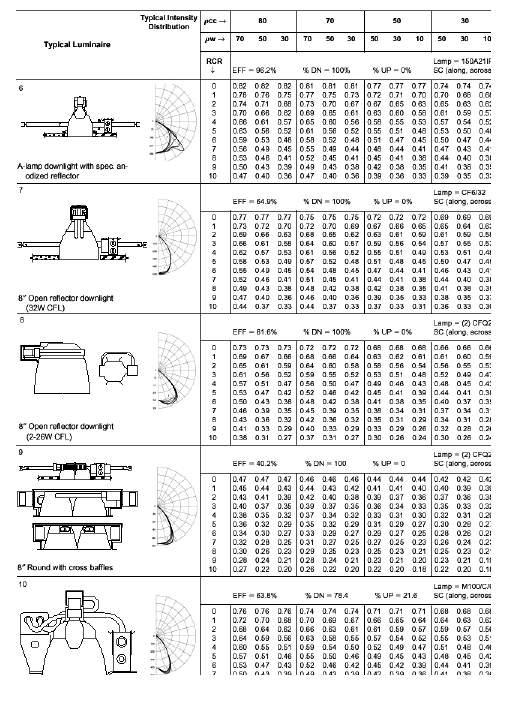Fig.6

to download tables for coefficient of utilization determination for different luminaires, please click on the link.

Step#8: Calculate Light Loss Factor (LLF)

There are two types of Light Loss Factor (LLF) as follows:

1. Recoverable,
2. Non-recoverable.

So, Total Light Loss Factor (LLF) is the product of the individual light loss factors, recoverable and non- recoverable.

Detailed description of the determination of the light loss factors can be found in the IESNA Lighting Handbook.

1- Recoverable LLF
This factor type will include the following sub-factors:

• Lamp Lumen Depreciation (LLD),
• Lamp Burnout Factor (LBO),
• Luminaire Dirt Depreciation Factor (LDD),
• Room Surface Dirt Depreciation Factor (RSDD),
• Area of workplane (AWP).

A- Lamp Lumen Depreciation
• The lamp lumen depreciation factor is the fraction of initial lumens at a specific time during the life of the lamp
• Lamp lumen depreciation comes from aging and dirt accumulation on lamps, reflectors, lenses and room surfaces.
• Most lighting designs base calculations on “maintained” as opposed to “initial” lamp lumens.

B- Lamp Burnout Factor
• If lamps are not replaced immediately after burnout, a lamp burnout factor should be applied to any analysis of the system.
• Unreplaced burned- out lamps will vary in quantity, depending on the kind of lamps and the relamping program used.
• This factor is simply the ratio of the number of lamps that would be burning o the total number of lamps in the system.

C- Luminaire Dirt Depreciation
Luminaire Dirt Depreciation Factor (LDD) depends on three (3) aspects of the situation as follows:

• The amount and type of dirt in the environment (a clean office environment compared to a dirty manufacturing facility),
• The type of luminaire used,
• The expected cleaning cycle for the equipment.

D- Room Surface Dirt Depreciation
Room Surface Dirt Depreciation Factor (RSDD) is influenced by:

• The amount of dirt in the environment,
• The room cavity ratio (proportions of the room),
• Type of lighting equipment used.

E- Area of Workplane
• Is the area of the entire workplane, which is typically the same as the floor area
• Illuminance will be greatest near the center of the room and slightly less toward the walls for a given uniform layout of luminaires

2- Non- Recoverable LLF
This factor type will include the following sub-factors:

• Luminaire Ambient Temperature Factor,
• Heat Extraction Thermal Factor,
• Voltage to Luminaire Factor,
• Ballast Factor,
• Ballast Lamp Photometer Factor,
• Equipment operating Factor,
• Lamp Position (Tilt) Factor,
• Luminaire Surface Depreciation Factor.

A- Luminaire Ambient Temperature
Variations in temperature, above those normally encountered in interiors, have little effect on the output of incandescent and high intensity discharge (HID) lamps, but can have a significant effect on light output of fluorescent lamps.

B- Heat Extraction Thermal Factor
• Heat extraction factor is the fractional lumen loss or gain due to airflow.
• Airflow has an effect on lamp temperature and lamp lumens especially those air handling fluorescent luminaires which are integrated with the HVAC system as a means of introducing or removing air from the room.

C- Voltage to Luminaire Factor
• High or low voltage at the luminaire will affect the lumen output of lamps.
• High voltage condition will increase the lumen output of lamps over their rated output.
• Low voltage condition will reduce the lumen output.
• The rate of change of lumen output with a voltage change varies with each light source, but has the greatest effect on incandescent lamps.

D- Ballast Factor
• Ballast used for a specific application is usually different from the ballast used to determine the rated lumen output for a lamp.
• Ballast factor corrects this difference to maintain the arc within the lamp.
• Ballast factor is the ratio of the lamp lumens generated on commercial ballasts to those generated on the test quality ballasts . The ballast factor for good quality fluorescent ballast is nominally is 0.95while electronic ballasts can have ballast factors ranging from 0.70 to 1.28.

E- Ballast Lamp Photometer Factor
• Ballast Lamp Photometer Factor adjusts the lumen output when a different lamp ballast combination is used other than the manufacturer’s set- up.
• Temperature effects within the luminaire may cause the lamp to operate at less than the rated output and should be considered in the determination of the luminaire’s

F- coefficient of utilization
Equipment Operating Factor Effects on the lumen output of lamps caused by the ballast, the lamp operating position and the effect of power reflected from the luminaire back onto the lamp are collectively incorporated into the equipment operating factor.

G- Lamp Position Factor
• Lumen output is sensitive to the lamp orientation especially for high intensity discharge (HID) lamps when they are tilted from their rated horizontal or vertical position.
• Lamp position factor adjusts the lumen output and is defined as the ratio of luminous flux in the given operating position to that in the test position.

H- Luminaire Surface Depreciation
• Luminaire surface depreciation results from adverse changes in metal, paint and plastic.
• components that result in permanently reduced light output.
• Luminaire surface depreciation factor adjusts light output to original reflectance.

Example #5:

Assuming the following:

1- Recoverable Factors:

• Lamp Lumen Depreciation (LDD) 0.90
• Lamp Burnout Factor (LBO) 1.00
• Luminaire Dirt Depreciation Factor (LDD) 0.94
• Room Surface Dirt Depreciation Factor (RSDD) 0.96
2- Nonrecoverable Factors:

• Ballast Factor 0.93
• Other Non Recoverable Factors 1.00
Calculate lights loss factor (LLF).

LLF Total = Recoverable Factors x Nonrecoverable Factors

LLF
Total = 0.90 x 1.00 x 0.94 x 0.96 x 0.93 x 1.00

LLF
Total = 0.75 Total Light Loss Factor (LLF) is 0.75, which means that 25% (100%-75%) of the luminous flux that might otherwise reach the workplane is lost due to ballast factor, dirty luminaires, room surfaces, and aged lamps.

Note: you can use the criteria developed by IESNA for getting an approximate value for the LLF TOTAL ,you can download this Criteria by clicking on the link.

In the next Article, I will explain other steps of zonal cavity method by using IES procedure plus the spacing criteria . So, please keep following.

1.2.